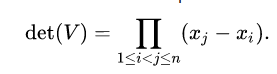# Vandermonde Determinant Function

Hi All,

I’ve been looking for an equivalent determinant function that computes the determinant of a Vandermonde Matrix. In short, the Vandermonde determinant scales better than a standard determinant due to the fact that the matrix is restricted.

Such a determinant is defined as,where `x` is a vector of length `n`.

I’ve written up a quick function that works, which is,

``````def vander_det(x: Tensor) -> Tensor:
""""
:param x: A Tensor of size [B,N]
"""
det = 1.
for j in reversed(range(x.shape[-1])):
for i in reversed(range(j)):
det *= (x[:,j] - x[:,i])
return det
``````

Although this works I’ve noticed that it actually scales worse than using `torch.linalg.det` (most likely due to the use a nested-loop. I was wondering if 1) There already exists a vandermonde-determinant like function within PyTorch (which I’ve missed) or 2) Is there a way to parallelize the nested loop?

Any help would be greatly apprecitated!

Thank you!Hi Alpha!

I doubt that your nested-loop version scales worse that `det()`. Your
version scales in time as `n**2` (just count the number of times through
the loop), while `det()` scales as `n**3`. Of course for moderate values
of `n` your python-loop version is indeed likely to be much slower than
`det()` because of the python, non-pipelined overhead.

Yes. With a little bit of monkeying around, you can perform the `n**2`
computation with pure, non-loop tensor operations:

``````>>> import torch
>>> torch.__version__
'1.9.0'
>>>
>>> _ = torch.manual_seed (2021)
>>>
>>> n = 5
>>>
>>> x = torch.randn (n)   # random x
>>>
>>> V = x.unsqueeze (1).expand (n, n)**torch.arange (n)   # vandermonde matrix
>>>
>>> torch.det (V)   # vandermonde determinant
tensor(2.2679)
>>>
>>> d = x - x.unsqueeze (1)   # "outer difference" -- x_j - x_i
>>>
>>> (torch.ones (n, n).tril() + d.triu (1)).prod()   # product of upper triangle of d  =  det (V)
tensor(2.2679)
``````

Best.

K. Frank

1 Like

Hi @KFrank!

Thanks for the quick and detailed response!

That’s what I thought but when I tested it the Vandermonde determinant scales bettter initially but for larger `N` performed worse. The Vandermonde det scaled approximately `N**2` but `torch.linalg.det` scaled better which doesn’t make sense to me because it’s an `N**3` operation, although that’s the naive scaling of it as it can be reduced.

What I’m probably doing is calculating the scaling wrong. The TL;DR of that is I just use `curve_fit` from `scipy` and fit a function of `f = a*x**b`. For Vandermonde is was about 2.1 and for `torch.linalg.det` it was like 1.4 (which doesn’t make sense at all, most likely because I’m calculating the scaling wrong). Perhaps it’d be better to `curve_fit` to logarithm of the runtime.

Thanks for the great example!